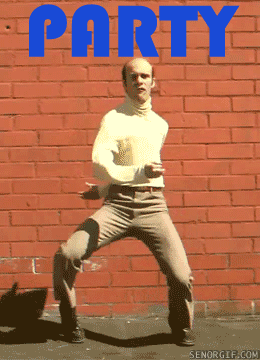# A 3+ YEAR ACCOMPLISHMENT FOR NS PARTY THIS FRIDAY!##A 3+ YEAR ACCOMPLISHMENT FOR NS PARTY THIS FRIDAY!

=D =D =D =D =D =D =D =D =D =D =D =D =D =D =D =D =D =D =D =D =D =D =D =D

Hey whats up guys...well, the community has now been up for 3+ years, almost 4 years so I'm throwing an all day party this upcoming Friday. Going to be custom events up the ying yang as well.

3+ years of awesome people and awesome times. =D =D GL HF

=D =D =D =D =D =D =D =D =D =D =D =D =D =D =D =D =D =D =D =D =D =D =D =DPermissions in this forum:
You cannot reply to topics in this forum